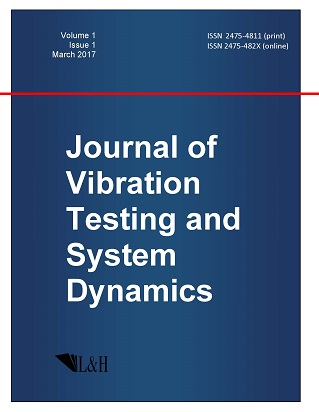ISSN: 2475-4811 (print)
ISSN: 2475-482X (online)
Journal of Vibration Testing and System Dynamics

C. Steve Suh (editor), Pawel Olejnik (editor),

Xianguo Tuo (editor)

Pawel Olejnik (editor)

Lodz University of Technology, Poland

Email: pawel.olejnik@p.lodz.pl

C. Steve Suh (editor)

Texas A&M University, USA

Email: ssuh@tamu.edu

Xiangguo Tuo (editor)

Sichuan University of Science and Engineering, China

Email: tuoxianguo@suse.edu.cn

On the Derivation of the Equations of Gravitation and Electrodynamics from the Generalized Least Action Principle and the Nonrelativistic Models of the Universe

Journal of Vibration Testing and System Dynamics 7(1) (2023) 39--47 | DOI:10.5890/JVTSD.2023.03.006

V.V. Vedenyapin, N.N. Fimin, A.A. Russkov, M.Yu. Voronina

Keldysh Institute of Applied Mathematics of RAS, Moscow, Miusskaya square 4, Russian Federation

Abstract

Of the Maxwell and Einstein equations in the framework of the Vlasov--Maxwell--Einstein equations from the classical, but more general principle of least action. The resulting derivation of the Vlasov--type equations gives the Vlasov--Einstein equations different from those proposed earlier. A method is proposed for the transition from kinetic equations to hydrodynamic--type equations. In the case of Hamiltonian mechanics, the transition to the Hamilton--Jacobi equation from the hydrodynamic consequences of the Liouville equation is possible, as was done already in quantum mechanics. Thus, in the nonrelativistic case, we obtain the Milne--McCrea{--type} solutions.

References

1.   Fock, V.A.G. (1959), Theory of Space, Time and Gravitation, Pergamon Press: London.
2.   Landau, L.D. and Lifshitz, E.M. (1962), The Classical Theory of Fields, McGraw-Hill Pergamon Press: Oxford.
3.   Wenberg, S. (1972), Gravitation and Cosmology: Principles and Applications of the General Theory of Relativity, John Wiley \& Sons: New York.
4.   Vlasov, A.A. (1966), Statistical Distribution Functions, Nauka: Moscow.
5.   Narlikar, J.V. (1993), Introduction to Cosmology, Cambridge University press: Cambridge.
6.   Vedenyapin, V.V. and Negmatov, M.A. (2012), On the derivation and classification of equations of the Vlasov and MHD type. Lagrange's identity and Godunov's form, Theoretical and Mathematical Physics, 170(3), 468-480.
7.   Vedenyapin, V.V., Negmatov, M.A., and Fimin N.N. (2017), Equations of the Vlasov and Liouville type, their microscopic, energetic and hydrodynamic consequences, Keldysh Institute of Applied Mathematics, 81(3), 45-82.
8.   Vedenyapin, V.V. and Negmatov, M.A. (2013), On the derivation and classification of equations of the Vlasov type and magnetohydrodynamics. Lagrange identity, Godunov form and critical mass, Sovremennaya Matematika. Fundamental'nye Napravleniya, 47, 5-17.
9.   Vedenyapin, V.V. (2001), Kinetic Boltzmann and Vlasov Equations, Fizmatlit: Moscow.
10.   Vedenyapin, V.V., Fimin N.N., and Chechetkin V.M. (2020), The system of Vlasov--Maxwell--Einstein-type equations and its nonrelativistic and weak relativistic limits, International Journal of Modern Physics D, 29(1), 1-23.
11.   Vedenyapin, V.V., Fimin N.N., and Chechetkin V.M. (2020), The properties of Vlasov--Maxwell--Einstein equations and its applications to cosmological models, The European Physical Journal Plus, 135(5), 1-14.
12.   Milne, E.A. (1935), Relativity, Gravitation and World--Structure, Clarendon Press: Oxford.